第十一讲 数据清洗

数据质量的准则

1、完整性：单条数据是否存在空值，统计的字段是否完善。
2、全面性：观察某一列的全部数值，比如平均值、最大值、最小值，根据常识判断是否有问题。如：数据定义、单位标识、数值本身。
3、合法性：数据的类型、内容、大小的合法性。如：存在非ASCII字符、性别未知、年龄过高等等。
4、唯一性：数据是否重复记录，行数据及列数据都要是唯一的。

1、完整性

df1.isnull().any() #判断哪列有空值

• 删除：删除数据缺失的记录
• 均值：使用当前列的均值（df[‘Age’].fillna(df[‘Age’].mean),inplace=True)）
• 高频：使用当前列出现频率最高的数据(age_max=df[‘Age’].value_counts().index df[‘Age’].fillna(age_max,inplace=True))

#删除全空的行
df.dropna(how='all',inplace=True)


2、全面性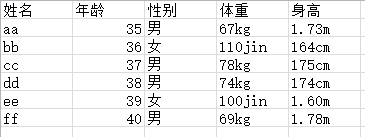import pandas as pd
rows_with_jins=df1['体重'].str.contains('jin').fillna(False)

for i,jin_row in df1[rows_with_jins].iterrows(): #iterrows()属于DataFrame（数据框）的遍历函数
weight=int(float(jin_row['体重'][:-3])/2) #这里有字符串到float再到int的转换
df1.at[i,'体重']='{}kg'.format(weight) #at[index，column]获取某个位置的值
#将m转化为cm，采取的方法是将包含cm的剔除
rows_with_cms=df1['身高'].str.contains('cm').fillna(False)
rows_with_ms = (1-rows_with_cms).astype(np.bool) #布尔型数组取反,将上句用"~"也同样能取反
for i,height in df1[rows_with_ms].iterrows():
m=float(height['身高'][:-1])*100 #这里的转换必须先将去掉m的字符串转换成float
df1.at[i,'身高']='{}cm'.format(m)
df1.to_excel('test.xlsx')


rows_with_jins: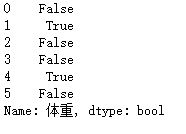iterrows() 横向遍历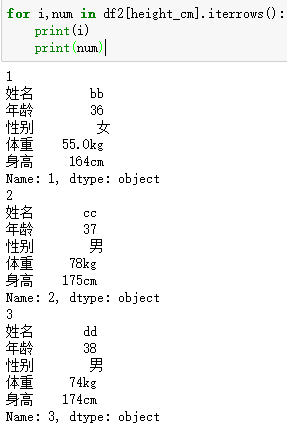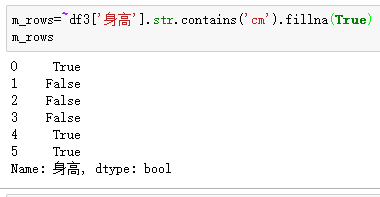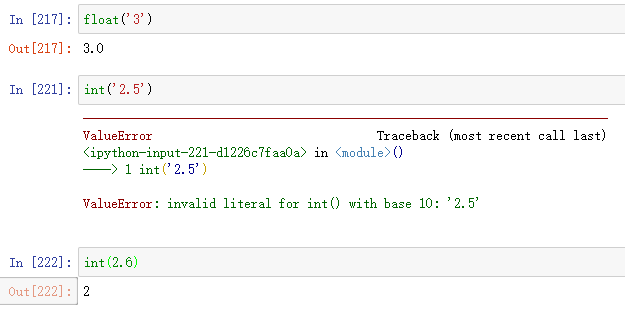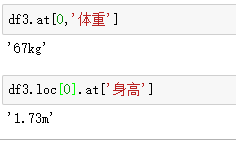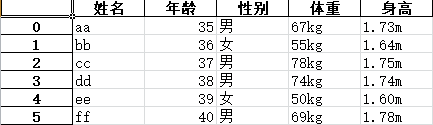ps.表是将cm转换成m，而将m转换成cm出现了以上一些问题，列出来以供学习和参考。

https://github.com/wesm/pydata-book

3.合理性

df['name'].replace({r'[^\x00-\x7F]+':''},regex=True, inplace=True)


4.唯一性

df[['first_name','last_name']]=df['name'].str.split(' ',expand=True)#expand=True参数将字符串拆分成多列，返回一个数据框,默认False。' '参数可省。
df.drop('name',axis=1,inplace=True) #删除源数据列


df4.drop_duplicates(['first_name','last_name'],inplace=True)